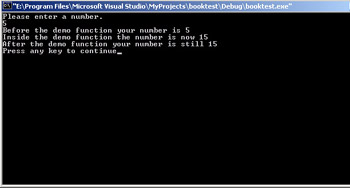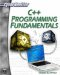# Passing Values by Reference

When you pass a value to a function you are really copying the contents of one variable to another variable—the other variable is the parameter of a function. This means the parameter is, essentially, a copy of the variable that was passed to the function. This means that you are not changing the value of the variable that is outside the function. That might sound confusing, so let’s look at an example that illustrates this concept.

### Example 4.5

Step 1: Place the following code into your favorite text editor.

`#include <iostream>  using namespace std;    void demo(float);    int main ()    { float num1; cout << "Please enter a number. \n"; cin >> num1; cout << "Before the demo function your number is "     << num1 << "\n"; demo(num1); cout << "After the demo function your number is    still" << num1 << "\n";  return 0;    } void demo(float number)  {  number = number * 4;  cout << "Inside the demo function the number is now " << number << "\n";  }`

Step 2: Compile this code.

Step 3: Run the code. You should see something similar to the image shown in Figure 4.5.Figure 4.5: Passing variables.

As you can see from this example, what occurs inside the function has no bearing on the values of the variables outside the program. When you call the demo function, you take the value that is in num1 and place that value in the function parameter number. Number is an entirely new variable occupying its own space in memory and is separate from num1. This is called passing a variable by value. It is the standard way that variables are passed in C++ (as well as in Java and several other languages).

There is another way to pass a variable. That is by reference. When you pass by reference you don’t simply copy the value of some variable to a parameter. What you pass is a reference to the variable’s address in memory. What that means is that the parameter of the function is NOT a new variable. It is simply a new name for the variable. Both the variable name and the parameter name refer to the same place in memory, they refer to the same value. You accomplish this by simply adding the &or address of operator to your function parameter. The & means address of and it refers to the address in memory. Let’s look at the previous example redone to pass-by reference.

### Example 4.6

Step 1: Write the following code in your favorite text editor.

`#include <iostream>  using namespace std; void demo(float &number); int main ()  {   float num1;   cout << "Please enter a number. \n";   cin >> num1;   cout << "Before the demo function your number is " << num1 << "\n";   demo(num1);   cout << "After the demo function your number is"    << num1 << "\n"; return 0; } void demo(float &number) {  number = number * 4;  cout << "Inside the demo function the number is now    <DS>" << number << "\n"; }`

Step 2: Compile the code.

Step 3: Run the code. You should see something like what is shown in Figure 4.6.Figure 4.6: Passing with the & operator.

Notice that this time when the value is changed inside the function, it is also changed in the variable outside the function. This is because you passed the address of that variable to the function. The parameter name was just an alias for the variable. Whatever you did inside the function was actually happening to the variable.C++ Programming Fundamentals (Cyberrookies)
ISBN: 1584502371
EAN: 2147483647
Year: 2005
Pages: 197
Authors: Chuck Easttom